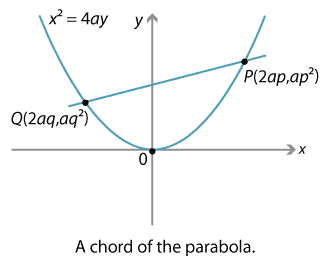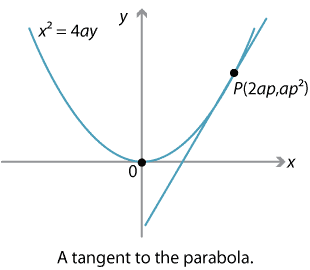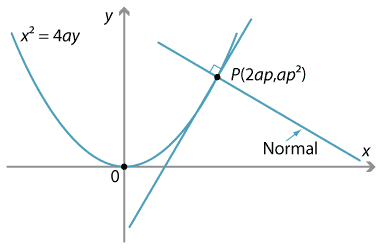### Chords, tangents and normals

#### Chords

Again, we consider the parabola $$x^2=4ay$$, for some $$a > 0$$, and the parametrisation

\begin{align*} x &= 2at\\ y &= at^2. \end{align*}

When we take two distinct points $$P,Q$$ on the parabola, we can join them to form a line segment called a chord. Sometimes we will refer to the line through $$P$$ and $$Q$$ as the chord.

Let the value of the parameter $$t$$ at the points $$P$$ and $$Q$$ be $$p$$ and $$q$$ respectively. Thus $$P$$ has coordinates $$(2ap, ap^2)$$ and $$Q$$ has coordinates $$(2aq, aq^2)$$.Detailed description of diagram

The gradient of the line $$PQ$$ is given by

$\dfrac{ap^2-aq^2}{2ap-2aq} = \dfrac{a(p^2-q^2)}{2a(p-q)}= \dfrac{p+q}{2}.$

So the gradient of the chord is the average of the values of the parameter. (One of the many reasons this parametrisation was chosen.)

Hence the equation of the chord is

$y-ap^2 = \dfrac{p+q}{2}(x-2ap),$

which rearranges to

$y = \dfrac{p+q}{2}x-apq.$

It is best to reconstruct this equation each time rather than memorise it.

A chord which passes through the focus of a parabola is called a focal chord. A given chord will be a focal chord if the point $$(0,a)$$ lies on it. Substituting these coordinates into the equation of the chord above we have

$a= \dfrac{p+q}{2}\times 0 -apq,$

which is equivalent to $$pq=-1$$. This gives us a simple condition to tell when the chord joining two points is a focal chord.

Thus $$PQ$$ is a focal chord if and only if $$pq=-1$$. The basic fact should be committed to memory.

#### Example

Suppose $$P(2ap, ap^2)$$ and $$Q(2aq,aq^2)$$ are two points lying on the parabola $$x^2=4ay$$.

Prove that, if $$OP$$ and $$OQ$$ are perpendicular, then $$pq=-4$$.

#### Solution

The gradient of $$OP$$ is $$\dfrac{ap^2}{2ap}= \dfrac{p}{2}$$ and similarly the gradient of $$OQ$$ is $$\dfrac{q}{2}$$. Since these lines are perpendicular, the product of their gradients is $$-1$$ and so $$\dfrac{p}{2}\times\dfrac{q}{2}=-1$$, which implies that $$pq= -4$$.

Note that, in this case, $$PQ$$ can never be a focal chord.

##### Exercise 15

Suppose $$P(2ap, ap^2)$$ and $$Q(2aq,aq^2)$$ are two points lying on the parabola $$x^2=4ay$$. Prove that, if $$PQ$$ is a focal chord, then the length of the chord $$PQ$$ is given by $$a\big(p+\dfrac{1}{p}\big)^2$$.

#### Tangents

We have seen that the gradient of the chord joining two distinct points $$P(2ap,ap^2)$$ and $$Q(2aq,aq^2)$$ on the parabola $$x^2=4ay$$ is $$\dfrac{1}{2}(p+q)$$.

If we now imagine the point $$Q$$ moving along the parabola to the point $$P$$, then the line through $$P$$ and $$Q$$ will become a tangent to the parabola at the point $$P$$. Putting $$q=p$$, we see that the gradient of this tangent is $$\dfrac{1}{2}(p+p) = p$$.Detailed description of diagram

We could also find the gradient of the tangent by differentiating

$y= \dfrac{x^2}{4a}$

and substituting $$x=2ap$$. In either case, the gradient of the tangent to $$x^2=4ay$$ at the point $$P(2ap,ap^2)$$ is $$p$$. (This is the main reason for this choice of parametrisation.)

Hence the equation of the tangent to the parabola at $$P$$ is given by

$y-ap^2= p(x-2ap) \ \implies \ y=px-ap^2.$

This formula is best derived each time it is needed rather than memorised.

#### Normals

The line through a point $$P$$ perpendicular to the tangent to the curve at $$P$$ is called the normal to the curve at $$P$$.

Since the normal and tangent are perpendicular, the product of their gradients is $$-1$$ and so the gradient of the normal at $$P(2ap, ap^2)$$ is $$-\dfrac{1}{p}$$. Hence the equation of the normal at $$P$$ is

$y-ap^2 = -\dfrac{1}{p}(x-2ap) \ \implies \ x+py = 2ap+ap^3.$

Once again, this is best derived each time rather than memorised.Detailed description of diagram

#### Example

Find the point of intersection of the tangents to the parabola $$x^2=4ay$$ at the points $$P(2ap,ap^2)$$ and $$Q(2aq,aq^2)$$. What can be said about this intersection point if the chord $$PQ$$ is a focal chord?

#### Solution

The equations of the tangents at $$P$$ and $$Q$$ are respectively $$y=px-ap^2$$ and $$y=qx-aq^2$$. Equating these we find

$px-ap^2=qx-aq^2 \ \implies \ x= a(p+q).$

Substituting back we find that the $$y$$-coordinate is $$y=apq$$ and so the point of intersection is $$\big(a(p+q), apq\big)$$.

Now, if $$PQ$$ is a focal chord, then $$pq=-1$$ and so the point of intersection becomes $${\big(a(p-\dfrac{1}{p}), -a\big)}$$. While the $$x$$-coordinate of this point can vary, the $$y$$-coordinate is always $$-a$$ and so the point of intersection always lies on the directrix.

##### Exercise 16

Find the point of intersection $$R$$ of the normals to the parabola $$x^2=4ay$$ at the points $$P(2ap, ap^2)$$ and $$Q(2aq, aq^2)$$.

Show that, if $$PQ$$ is a focal chord, then the point $$R$$ always lies on a certain parabola, and find the equation of this parabola.

Next page - Content - The reflection property of the parabola